# Ricci curvature

Jump to: navigation, search

## Definition

### In terms of Ricci curvature tensor

Let$M$ be a differential manifold and$g$ a Riemannian metric on$M$. The Ricci curvature on$g$ is a function from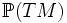$\mathbb{P}(TM)$ (the set of tangent directions) to$\R$ (real numbers) that associates to a particular tangent direction the value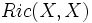$Ric(X,X)$ where$X$ is a unit tangent vector in that direction.

### In terms of sectional curvature

Another way of defining the Ricci curvature is in terms of the sectional curvature. Let$(M,g)$ be a Riemannian manifold,$p \in M$ and$X$ a unit tangent vector at$p$. Let$e_1, e_2, \ldots e_n$ be an orthonormal basis at$p$ such that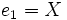$e_1 = X$. Then the Ricci curvature of$X$ is defined as: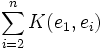$\sum_{i=2}^n K(e_1,e_i)$

By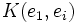$K(e_1,e_i)$ is meant the sectional curvature of the plane spanned by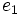$e_1$ and$e_i$.

### In terms of Riemann curvature tensor

We now define the Ricci curvature directly in terms of the Riemann curvature tensor, and this definition explains both the above definitions. The Ricci curvature at a point, for a tangent direction with unit tangent vector$X$, is defined as: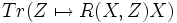$Tr(Z \mapsto R(X,Z)X)$

or equivalently, if we choose an orthonormal basis with$X=e_1$ as: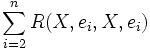$\sum_{i=2}^n R(X,e_i,X,e_i)$

This gives the above two definitions.

## Facts

### Ricci curvature determines Ricci curvature tensor

This is analogous to how the sectional curvature determined the Riemann curvature tensor

Further information: Ricci curvature determines Ricci curvature tensor

This rests on two observations:

• The Ricci curvature tensor is symmetric
• A symmetric bilinear form is completely determined by the values it takes on pairs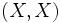$(X,X)$ because of the identity: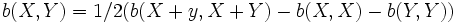$b(X,Y) = 1/2(b(X+y,X+Y) - b(X,X) - b(Y,Y))$

It is also easy to see that:

• If the Ricci curvature is constant on all unit tangent vectors at a point, then the Ricci curvature tensor at that point is that constant times the Riemannian metric restricted to that tangent space

The converse is also true.

• Thus, the Ricci curvature is constant on all unit tangent vectors at all points if and only if the Ricci curvature tensor is that constant times the Riemannian metric. Such Riemannian metrics are termed Einstein metrics and the constant of proportionality is termed the cosmological constant.

### Ricci curvature for constant-curvature metrics

The Ricci curvature at a unit tangent vector has been defined as a sum of sectional curvatures for an orthonormal basis involving that unit tangent vector. In particular, if the sectional curvature is constant for all tangent planes at the given point, then the Ricci curvature is$(n-1)$ times that constant. Thus, any constant-curvature metric is an Einstein metric and the cosmological constant is$(n-1)$ times the constant curvature.